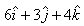Courses

# Test: Gauss Law

## 10 Questions MCQ Test Physics Class 12 | Test: Gauss Law

Description
This mock test of Test: Gauss Law for Class 12 helps you for every Class 12 entrance exam. This contains 10 Multiple Choice Questions for Class 12 Test: Gauss Law (mcq) to study with solutions a complete question bank. The solved questions answers in this Test: Gauss Law quiz give you a good mix of easy questions and tough questions. Class 12 students definitely take this Test: Gauss Law exercise for a better result in the exam. You can find other Test: Gauss Law extra questions, long questions & short questions for Class 12 on EduRev as well by searching above.
QUESTION: 1

### The Gaussian surface for a point charge will be

Solution:

A point charge is single dimensional. The three dimensional imaginary enclosed surface of a point charge will be sphere.

QUESTION: 2

### At what point is the electric field intensity due to a uniformly charged spherical shell is maximum?

Solution:

This is because when a charge is given to a hollow sphere that is a spherical shell then all charges will reside on its surface and as we know that electric field is directly proportional to given charge . so according to this concept of gauss’s law we can say about this point.

QUESTION: 3

### A charge q is placed at the centre of the open end of cylindrical vessel whose height is equal to its radius. The electric flux of electric field of charge q through the surface of the vessel is

Solution:

Given that, A charge q is placed at the centre of open end Q a cylindrical vessel,we have to find the flux through the surface of the vessel.
so, when charge Q is placed at the centre of open end of a cylindrical vessel then only half of the charge will contribute to the flux, because half will lie inside the surface and half will lie outside the surface.
so, flux through the surface of vessel is q/2ε0

QUESTION: 4

Which of the following quantity is a scalar?

Solution:

Electric flux is a scalar quantity. It is a scalar because it is the dot product of two vector quantities, electric field and the perpendicular differential area.

QUESTION: 5

If an excess charge is placed on an isolated conductor, then, that amount of charge

Solution:

An isolated conductor is any metal object not connected to or not in contact with any other conductor . one of its common properties is that there is no charge of any nature within the surface of the conductor. All charge, if any, always resides on the outer surface only.thus , if we place any amount of charge, it would tend to reside on its surface.

QUESTION: 6

The charge enclosed by a spherical Gaussian surface is 8.85 X 10-8 C.What will be the electric flux through the Surface?

Solution:

we know that the flux linked with a Gaussian surface is given as
Φ = E.ds = q/ε0
here,
q = 8.85x10-8 C
ε0 = 8.85x10-12 F/m
so,
Φ = 8.85x10-8 / 8.85x10-12
thus, flux will be
Φ = 104 Nm2/C-1

QUESTION: 7

If the electric field is given by; calculate the electric flux through as surface of area 10 units lying in y-z plane.​

Solution:

As surface of area lies in the Y-Z plane, thus its area vector points in X direction i.e.   A=10i
Electric flux    ϕ=E.A
∴   ϕ=(6i+3j​+4k).(10i)
⟹   ϕ=6×10=60 unit

QUESTION: 8

The flux associated with a spherical surface is 104 N m2C-1.  What will be the flux, if the radius of the spherical shell is doubled?​

Solution:

If the radius of the spherical shell is double then also flux associated with spherical surface remain unchanged because the charge enclosed in system always remains unchanged.

QUESTION: 9

The Gaussian surface for a line charge will be

Solution:

A line charge can be visualized as a rod of electric charges. The three dimensional imaginary enclosed surface of a rod can be a cylinder.

QUESTION: 10

The electric field intensity due to a sphere (solid or hollow) at an external point varies as-​

Solution:

In case of a sphere (solid/hollow), electric intensity (E) at an external point varies as 1/r2, where r is the distance of the point from the centre of the sphere. In case of a line charge, E o 1/r and in case of an infinite plane sheet of charge, E does not depend upon r.